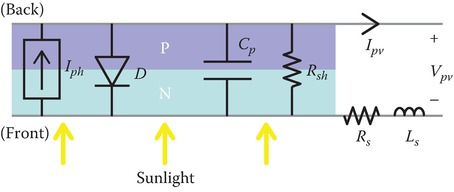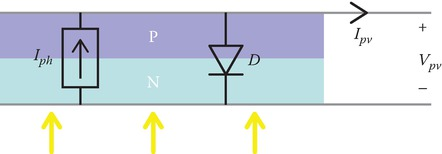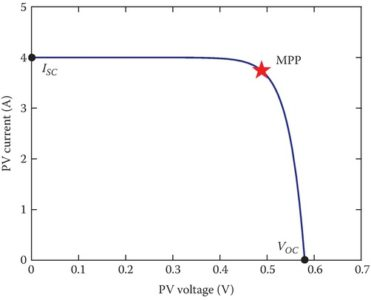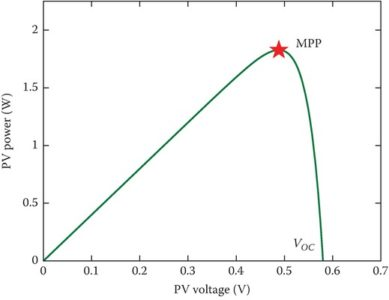Home / Renewable Energy / Photovoltaic (PV) Cell: Working & Characteristics

# Photovoltaic (PV) Cell: Working & Characteristics

Want create site? Find Free WordPress Themes and plugins.

Photovoltaic (PV) cells, or solar cells, are semiconductor devices that convert solar energy directly into DC electric energy. In the 1950s, PV cells were initially used for space applications to power satellites, but in the 1970s, they began also to be used for terrestrial applications.

Today, PV cells are used to provide power in a wide variety of applications, including grid-connected systems (e.g., utility-scale and residential), remote buildings, outdoor traffic-related equipment, and satellites.

An example of a roof-mounted residential grid-connected PV system providing power to a campus building is shown in Figure 1.FIGURE 1 Roof-mounted grid-connected PV system at Ulsan National Institute of Science and Technology in Ulsan, South Korea.

PV cells can be made from many different types of materials and be using a range of fabrication techniques. As shown in Figure 1, the major categories of PV materials are crystalline silicon (Si), thin film, multi-junction, and various emerging technologies like dye-sensitized, perovskite, and organic PV cells.

Today, there is a significant amount of research that focuses on both increasing efficiency and decreasing manufacturing cost. However, the most dominant type of PV cell used in large-scale applications is still crystalline silicon, which is the same basic technology as used in the 1970s. This is partially due to the high availability of low-cost silicon PV panels that have prevented new and emerging cell types from gaining a significant presence in the PV market.

PV materials and fabrication techniques have made significant headway in the last 15 years and a shift in the PV cell type may be on the horizon, but, for now, crystalline silicon is still the dominant cell type. This section will introduce and detail the basic characteristics and operating principles of crystalline silicon PV cells as some considerations for designing systems using PV cells.

## Photovoltaic (PV) Cell Basics

A PV cell is essentially a large-area p–n semiconductor junction that captures the energy from photons to create electrical energy.

At the semiconductor level, the p–n junction creates a depletion region with an electric field in one direction. When a photon with sufficient energy hits the material in the depletion region, the energy from the photon excites a valence electron into the conduction band, leaving a hole in the valence band. Due to the electric field in the depletion region, the electron and hole will travel in opposite directions and generate a net current. This process of a photon generating an electron-hole pair is shown in Figure 2. This generated current over the voltage generated by the semiconductor junction allows the PV cell to generate DC power.FIGURE 2 Process of a photon generating an electron-hole pair in a PV cell.

There are two basic types of crystalline silicon cells: mono-crystalline (m-c) and poly-crystalline (p-c).

The m-c cells have one uniform lattice through the entire cell and allow electronics to flow easily through the materials, while p-c cells have multiple crystalline structures, or grains, which can impede electron flow. Thus, p-c cells tend to have lower conversion efficiency than m-c cells, but they are slightly cheaper to manufacture.

Figure 3 shows images of an m-c and p-c PV cell close-up, where the m-c material structure is uniform but the p-c materials have many different grain regions. Both m-c and p-c cells are widely used in PV panels and in PV systems today.FIGURE 3 A PV cell with (a) a mono-crystalline (m-c) and (b) poly-crystalline (p-c) structure.

## Photovoltaic (PV) Cell Components

The basic structure of a PV cell can be broken down and modeled as basic electrical components. Figure 4 shows the semiconductor p–n junction and the various components that make up a PV cell.

The photon-to-electron flow process explained previously can be modeled as a current source, Iph, where the generated current depends on the intensity of the light hitting the cell. The p–n semiconductor junction is modeled as a diode, D, with the direction as shown in Figure 4.

The current source and diode make up the ideal model of a PV cell, but in real life, there are additional parasitic components. The p–n junction will have associated parallel capacitance, Cp, and parallel resistance (also called shunt resistance), Rsh, while the wire leads attached to the PV cell will have associated series resistance, Rs, and series inductance, Ls. These parasitic components are often ignored when only a simple representation of a PV cell or panel is needed, but they should be taken into consideration when more accurate modeling is required.FIGURE 4 PV cell basic structure electrical model components with parasitic components.

## PV Operating Characteristics

While there are many environmental factors that affect the operating characteristics of a PV cell and its power generation, the two main factors are solar irradiance G, measured in W/m2, and temperature T, measured in degree Celsius (°C). The relation between these two factors and the PV operating characteristics can be modeled mathematically. First, we examine the ideal model that consists of just the photocurrent source Iph and a diode, as it is shown in Figure 5.FIGURE 5 Ideal PV model with a current source and diode.

The photocurrent Iph depends on both the irradiance and temperature according to

$\begin{matrix} {{I}_{ph}}(G,T)=\left[ {{I}_{scn}}+Ki\left( T-{{T}_{n}} \right) \right]\frac{G}{{{G}_{n}}} & {} & \left( 1 \right) \\\end{matrix}$

where

Iscn is the nominal short-circuit current

Ki is the current temperature coefficient

Gn is the nominal solar irradiance, which is typically 1000 W/m2

Tn is the nominal cell temperature, which is typically 25 °C

These values can be determined from the ratings listed for commercial PV cells or panels. Also, the current Id and voltage Vd of the diode are expressed by an exponential relation, and they are represented as

$\begin{matrix} {{I}_{d}}(T,{{V}_{d}})={{I}_{s}}(T)\left[ \exp \left( \frac{{{V}_{d}}}{\alpha {{V}_{t}}(T)} \right) \right] & {} & \left( 2 \right) \\\end{matrix}$

where

Is is the diode saturation current

a is the diode ideality constant

Vd is the diode voltage

Vt is the thermal voltage of the semiconductor junction

The diode saturation current depends on temperature and can be defined as

$\begin{matrix} {{I}_{s}}(T)=\frac{{{I}_{scn}}+{{K}_{i}}\left( T-{{T}_{n}} \right)}{\exp \left( \frac{{{V}_{ocn}}+{{K}_{v}}\left( T-{{T}_{n}} \right)}{\alpha {{V}_{t}}(T)} \right)} & {} & \left( 3 \right) \\\end{matrix}$

where

Iscn is the nominal short-circuit current

Ki is the current temperature coefficient

Tn is the nominal cell temperature

Vocn is the nominal open-circuit voltage

Kv is the voltage temperature coefficient

a is the diode ideality factor

Vt is the thermal voltage

Note that the diode voltage Vd  is the same as the PV voltage Vpv for the ideal model. Also, the thermal voltage Vt depends on temperature T and is defined by

$\begin{matrix} {{V}_{t}}(T)=\frac{kT}{q}{{N}_{s}} & {} & \left( 4 \right) \\\end{matrix}$

where

k is Boltzmann’s constant (approximately 1.3807 × 10−23 J·K−1)

q is the electron charge (1.60217662 × 10−19 C)

Ns is the number of PV cells in series

Using Kirchhoff’s circuit laws, the relation between the PV current Ipv and PV voltage Vpv for the ideal PV model is

$\begin{matrix} {{I}_{pv}}={{I}_{ph}}(G,T)-{{I}_{d}}(T,{{V}_{pv}}) & {} & \left( 5 \right) \\\end{matrix}$

where the photocurrent Iph is defined in (1) and the diode current Id is defined in (2). Based on the PV current Ipv equation, given in (5), it is clear that the PV output current is related to the solar irradiance G and temperature T.

Given the solar irradiance and temperature, this explicit equation in (5) can be used to determine the PV current for a given voltage. These equations can also be rearranged using basic algebra to determine the PV voltage based on a given current.

## Photovoltaic (PV) Cell I-V Curve

The I–V curve of a PV cell is shown in Figure 6. The star indicates the maximum power point (MPP) of the I–V curve, where the PV will produce its maximum power.

At voltages below the MPP, the current is a relative constant as voltage changes such that it acts similar to a current source.

At voltages above the MPP, the voltage is relatively constant as current changes such that it acts similar to a voltage source.

The open-circuit voltage of a PV is the voltage when the PV current is 0 A, and it is labeled as VOC in Figure 6. The short-circuit current is the current when the PV voltage is 0 V, labeled as ISC.

These parameters are often listed on the rating labels for commercial panels and give a sense for the approximate voltage and current levels to be expected from a PV cell or panel.FIGURE 6 I–V curve for an example PV cell (G = 1000 W/m² and T = 25 °C; VOC: open-circuit voltage; ISC: short-circuit current).

## Photovoltaic (PV) Cell P-V Curve

Based on the I–V curve of a PV cell or panel, the power–voltage curve can be calculated. The power–voltage curve for the I–V curve shown in Figure 6 is obtained as given in Figure 7, where the MPP is the maximum point of the curve, labeled with a star.

The I–V curve and power–voltage curve showed are under a specific irradiance and temperature condition. Over a day, both the irradiance and temperature will change, sometimes gradually (minutes to hours) and sometimes quickly (seconds), for example, due to passing clouds. Think of the I–V curves as the characteristics for just an instant of time.FIGURE 7 Power–voltage curve, for example, PV cell under a specific constant irradiance and temperature condition (i.e., G = 1000 W/m2 and T = 25 °C; VOC: open-circuit voltage).

## Effects of Solar Irradiance and Temperature Changes on a PV Cell I–V Curve

As irradiance and temperature change, the I–V curve will also change, as shown in Figure 8.

The irradiance is directly proportional to the current characteristics. As the irradiance increases, the short-circuit current and MPP current will also increase.

Conversely, the temperature is inversely proportional to the voltage characteristics. As the temperature increases, the open-circuit voltage (VOC) and MPP voltage will decrease.

According to these trends, a cell will produce the most power when the sunlight intensity is high but the temperature is low. However, these kinds of environmental conditions are not common, as higher-intensity light hitting an object tends to increase its temperature at the same time.FIGURE 8 Effects of (a) solar irradiance and (b) temperature changes on a PV’s I–V curve.

Did you find apk for android? You can find new Free Android Games and apps.